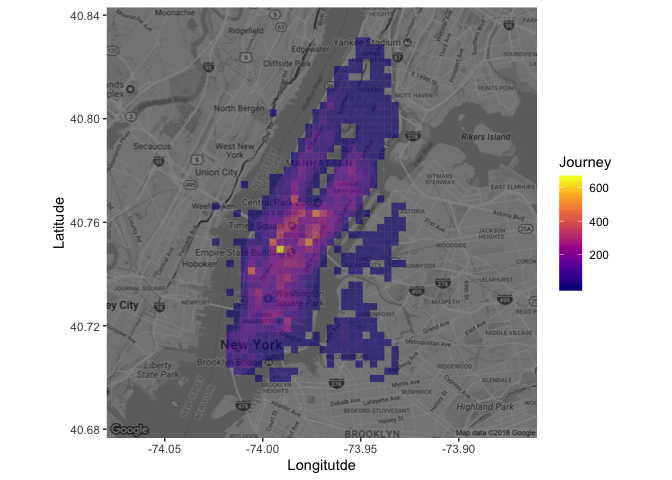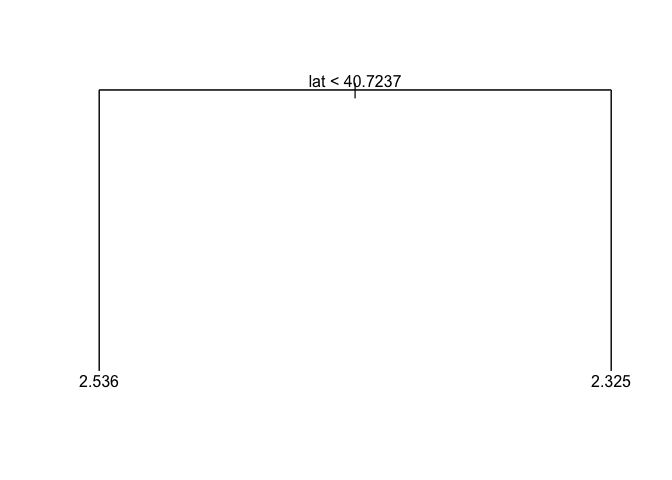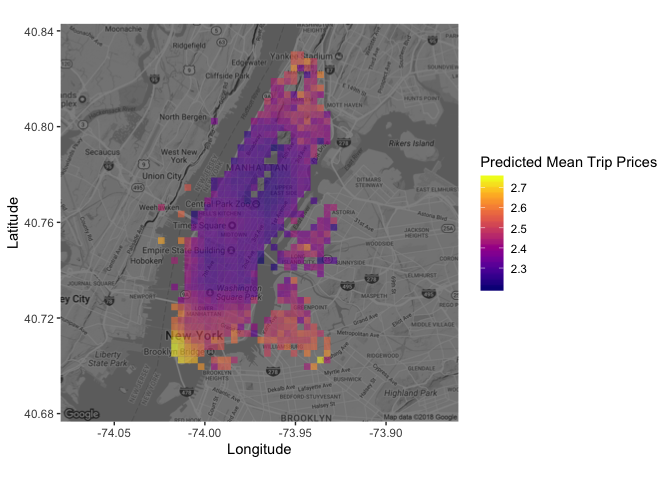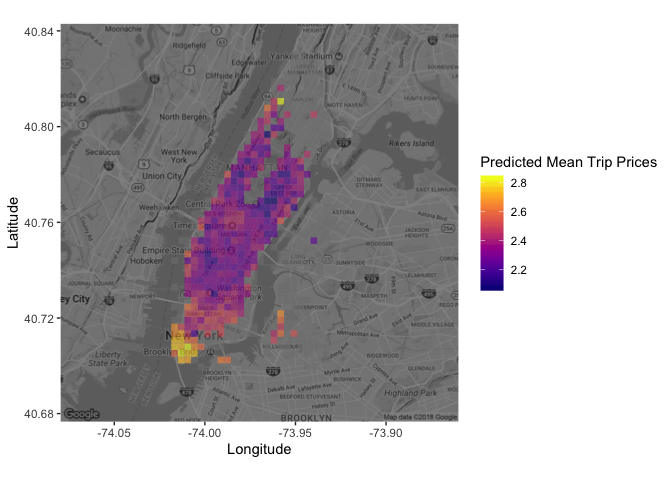## 1. 49999 New York taxi trips

To drive a yellow New York taxi, one has to hold a “medallion” from the city’s Taxi and Limousine Commission. Recently, one of those changed hands for over one million dollars, which shows how lucrative the job can be. But this is the age of business intelligence and analytics! Even taxi drivers can stand to benefit from some careful investigation of the data, guiding them to maximize their profits. In this project, I am analysing a random sample of 49999 New York journeys made in 2013 and using regression trees and random forests to predict the value of fares and tips, based on location, date and time. Let’s start by taking a look at the data!

# Loading the tidyverse
library(tidyverse)

# Reading in the taxi data

# Taking a look at the first couple of rows in taxi
head(taxi)
## # A tibble: 6 x 7
##   medallion pickup_datetime     pickup_longitude pickup_latitude
##   <chr>     <dttm>                         <dbl>           <dbl>
## 1 4D24F4D8… 2013-01-13 10:23:00            -73.9            40.8
## 2 A49C37EB… 2013-01-13 04:52:00            -74.0            40.7
## 3 1E4B72A8… 2013-01-13 10:47:00            -74.0            40.8
## 4 F7E4E943… 2013-01-13 11:14:00            -74.0            40.7
## 5 A9DC75D5… 2013-01-13 11:24:00            -74.0            40.8
## 6 19BF1BB5… 2013-01-13 10:51:00            -74.0            40.8
## # … with 3 more variables: trip_time_in_secs <dbl>, fare_amount <dbl>,
## #   tip_amount <dbl>

## 2. Cleaning the taxi data

As we can see above, the taxi dataset contains the times and price of a large number of taxi trips. Importantly we also get to know the location, the longitude and latitude, where the trip was started.

# Renaming the location variables, dropping any journeys with zero fares and zero tips,
# and creating the total variable as the log sum of fare and tip
taxi <- taxi %>%
rename(long = pickup_longitude, lat = pickup_latitude) %>%
filter(fare_amount > 0 | tip_amount > 0) %>%
mutate(total = log(fare_amount + tip_amount))

## 3. Zooming in on Manhattan

While the dataset contains taxi trips from all over New York City, the bulk of the trips are to and from Manhattan, so let’s focus only on trips initiated there.

# Reducing the data to taxi trips starting in Manhattan
taxi <- taxi  %>%
filter(between(lat, 40.70, 40.83),
between(long, -74.025, -73.93))

## 4. Where does the journey begin?

It’s time to draw a map! We’re going to use the excellent ggmap package together with ggplot2 to visualize where in Manhattan people tend to start their taxi journeys.

GGMAP: D. Kahle and H. Wickham. ggmap: Spatial Visualization with ggplot2. The R Journal, 5(1), 144-161. URL http://journal.r-project.org/archive/2013-1/kahle-wickham.pdf

# Loading in ggmap and viridis for nice colors
library(ggmap)
library(viridis)

# Retrieving a stored map object

# Drawing a density map with the number of journey start locations
ggmap(manhattan, darken = 0.5) +
scale_fill_viridis(option = 'plasma') +
geom_bin2d(data = taxi, aes(long, lat), bins = 60, alpha = 0.6) +
labs(x = 'Longitutde', y = 'Latitude', fill = 'Journey')## 5. Predicting taxi fares using a tree

The map showed that the journeys are highly concentrated in the business and tourist areas.

We’re now going to use a regression tree to predict the total fare with lat and long being the predictors. The tree algorithm will try to find cutpoints in those predictors that results in the decision tree with the best predictive capability.

# Loading in the rpart package
library(tree)

# Fitting a tree to lat and long
fitted_tree <- tree(formula = total ~ lat + long,
data = taxi)

# Draw a diagram of the tree structure
plot(fitted_tree)
text(fitted_tree)## 6. It’s time. More predictors.

The tree above looks a bit frugal, it only includes one split: It predicts that trips where lat < 40.7237 are more expensive, which makes sense as it is downtown Manhattan. But that’s it. It didn’t even include long as tree deemed that it didn’t improve the predictions.

Let’s start by adding some more predictors related to the time the taxi trip was made.

# Loading in the lubridate package
library(lubridate)

# Generate the three new time variables
taxi <- taxi %>%
mutate(hour = hour(pickup_datetime),
wday = wday(pickup_datetime, label = TRUE),
month = month(pickup_datetime, label = TRUE))

head(taxi)
## # A tibble: 6 x 11
##   medallion pickup_datetime      long   lat trip_time_in_se… fare_amount
##   <chr>     <dttm>              <dbl> <dbl>            <dbl>       <dbl>
## 1 4D24F4D8… 2013-01-13 10:23:00 -73.9  40.8              600         8
## 2 A49C37EB… 2013-01-13 04:52:00 -74.0  40.7              840        18
## 3 1E4B72A8… 2013-01-13 10:47:00 -74.0  40.8               60         3.5
## 4 F7E4E943… 2013-01-13 11:14:00 -74.0  40.7              720        11.5
## 5 A9DC75D5… 2013-01-13 11:24:00 -74.0  40.8              240         6.5
## 6 19BF1BB5… 2013-01-13 10:51:00 -74.0  40.8              540         8.5
## # … with 5 more variables: tip_amount <dbl>, total <dbl>, hour <int>,
## #   wday <ord>, month <ord>

## 7. One more tree!

Let’s try fitting a new regression tree where we include the new time variables.

# Fitting a tree with total as the outcome and
# lat, long, hour, wday, and month as predictors
fitted_tree <- tree(formula = total ~ lat + long + hour + wday + month,
data = taxi)

# draw a diagram of the tree structure
plot(fitted_tree)
text(fitted_tree)# Summarizing the performance of the tree
summary(fitted_tree)
##
## Regression tree:
## tree(formula = total ~ lat + long + hour + wday + month, data = taxi)
## Variables actually used in tree construction:
##  "lat"
## Number of terminal nodes:  2
## Residual mean deviance:  0.3041 = 13910 / 45760
## Distribution of residuals:
##     Min.  1st Qu.   Median     Mean  3rd Qu.     Max.
## -1.61900 -0.37880 -0.04244  0.00000  0.32660  2.69900

## 8. One tree is not enough

The regression tree has not changed after including the three time variables. This is likely because latitude is still the most promising first variable to split the data on, and after that split, the other variables are not informative enough to be included. A random forest model, where many different trees are fitted to subsets of the data, may well include the other variables in some of the trees that make it up.

# Loading in the randomForest package
library(randomForest)

# Fitting a random forest
fitted_forest <- randomForest(formula = total ~ lat + long + hour + wday + month,
data = taxi,
ntree = 80, sampsize = 10000)

# Printing the fitted_forest object
fitted_forest
##
## Call:
##  randomForest(formula = total ~ lat + long + hour + wday + month,      data = taxi, ntree = 80, sampsize = 10000)
##                Type of random forest: regression
##                      Number of trees: 80
## No. of variables tried at each split: 1
##
##           Mean of squared residuals: 0.2996623
##                     % Var explained: 2.81

## 9. Plotting the predicted fare

Now, let’s take a look at the predictions of fitted_forest projected back onto Manhattan.

# Extracting the prediction from fitted_forest
taxi$pred_total <- fitted_forest$predicted

# Plotting the predicted mean trip prices from according to the random forest
ggmap(manhattan, darken = 0.5) +
scale_fill_viridis(option = 'plasma') +
stat_summary_2d(data = taxi, aes(x = long, y = lat, z = pred_total), bins = 60, alpha = 0.6, fun = mean) +
labs(x = 'Longitude', y = 'Latitude', fill = 'Predicted Mean Trip Prices')## 10. Plotting the actual fare

Looking at the map with the predicted fares we see that fares in downtown Manhattan are predicted to be high, while midtown is lower. Let’s compare the map with the predicted fares with a new map showing the mean fares according to the data.

# Function that returns the mean *if* there are 10 or more datapoints
mean_if_enough_data <- function(x) {
ifelse( length(x) >= 20, mean(x), NA)
}

# Plotting the mean trip prices from the data
ggmap(manhattan, darken = 0.5) +
scale_fill_viridis(option = 'plasma') +
stat_summary_2d(data = taxi, aes(x = long, y = lat, z = total), bins = 60, alpha = 0.6, fun = mean_if_enough_data) +
labs(x = 'Longitude', y = 'Latitude', fill = 'Predicted Mean Trip Prices')